•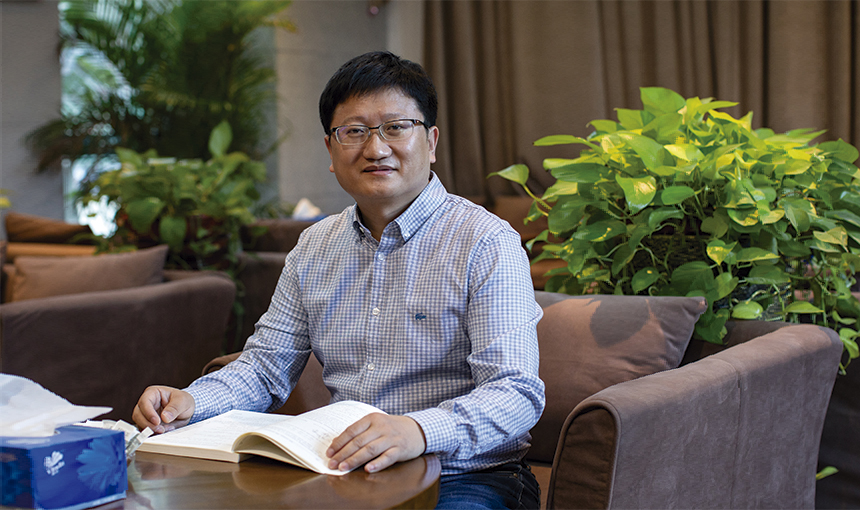2020 Second Prize of National Natural Science Award is awarded to Prof. Tian Ye for his extraordinary achievements in the Congruent number problem and arithmetic of L-function.
The congruent number problem, originated in an Arab manuscript dated A.C. 972, is one of the oldest unsolved major problems in number theory, and possibly in the whole of mathematics. It is closely related to the Birch and Swinnerton-Dyer conjecture, one of the seven Millennium Prize Problems.

# Prof. Tian Ye Awarded with 2020 Second Prize of National Natural Science Award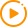Watch Video

11 10, 2021

2020 Second Prize of National Natural Science Award is awarded to Prof. Tian Ye for his extraordinary achievements in the Congruent number problem and arithmetic of L-function.

The congruent number problem, originated in an Arab manuscript dated A.C. 972, is one of the oldest unsolved major problems in number theory, and possibly in the whole of mathematics. It is closely related to the Birch and Swinnerton-Dyer conjecture, one of the seven Millennium Prize Problems. A positive integer is congruent if it is the area of a right-angled triangle, all of whose sides have rational length. The congruent number problem is the problem of deciding which positive numbers are congruent numbers and finding the corresponding right-angled triangle for a congruent number. Predicted by the BSD conjecture, all positive integer congruent to 5, 6 and 7 modulo 8 are conjectured to be congruent numbers. At 1952, the German mathematician K. Heegner verified this conjecture for square-free positive integers with only one odd prime factor.

In 2012, Prof. Tian Ye proved that there are infinitely many congruent numbers with any given prime factors, moreover, the BSD conjecture for the corresponding congruent elliptic curve hold. In the PNAS review, member of the Royal Society, Prof. John Coates from Cambridge University commented that Tian’s work is an important milestone in the history of this ancient problem. In addition, Prof. Tian Ye obtained important achievements on Gross-Zagier formula, twisted Fermat equations, the variation of rational points on elliptic curve under quadratic twists and Kummer extentions, and so on.

Prof. Tian Ye received his doctorate from Columbia University in 2003, supervised by Professor Zhang Shouwu. Then he served as a member of the Princeton Institute of advanced studies, and a postdoctoral fellow at McGill University in Canada. In 2006, he joined the Academy of Mathematics and Systems Science of the Chinese Academy of Sciences. His research field is mainly in number theory and arithmetic geometry. Due to the breakthrough work on the congruent number problem, he won the 2013 Morningside Gold Prize of Mathematics and the 2013 Ramanujan Prize.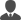Contacts: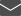E-mail: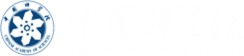•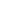Facebook
•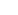Twitter
•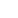Instagram
•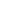Snapchat
•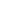LinkedIn
•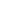YouTube
•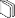Social Media Directory